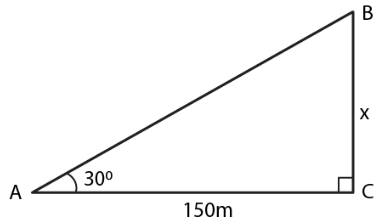Guru

# The angle of elevation of the top of a tower from a point on the ground and at a distance of 150 m from its foot is 300. Find the height of the tower correct to one place of decimal.

• 0

sir this is the question from the book -ML aggarwal( avichal publication) class 10th , chapter20 , heights and distances
we have the data that :- The angle of elevation of the top of a tower from a point on the ground and at a distance of 150 m from its foot is 300.
Now we have to find the  height of the tower correct to one place of decimal.
This is a very important question
question no 2 , heights and distances , ICSE board

Share

1. Consider BC as the tower and A as the point on the ground such that

∠A = 300 and AC = 150 mTake x m as the height of the tower

We know that

tan θ = BC/AC

Substituting the values

tan 300 = x/150

By cross multiplication

1/√3 = x/150

So we get

x = 150/√3

Multiplying and dividing by √3

x = (150 × √3)/ (√3 × √3)

By further calculation

x = 150√3/ 3 = 50√3 m

Substituting the value of √3

x = 50 (1.732)

x = 86.600 m

x = 86.6 m

• 0